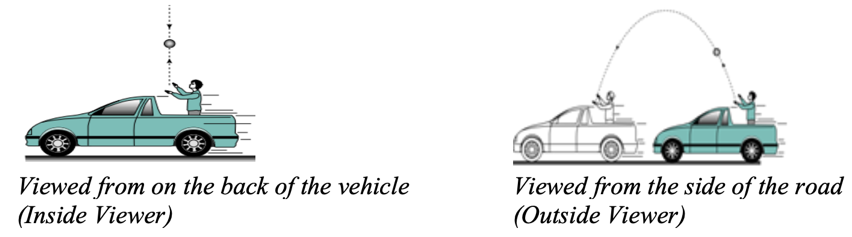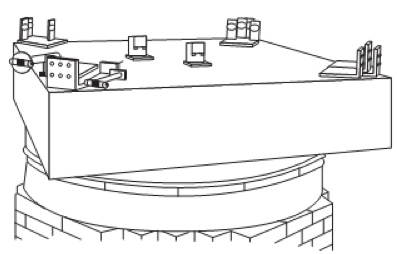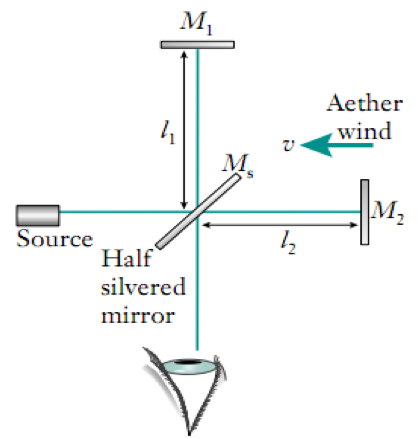# M7-S10: Einstein's Postulates of Special Relativity• Frame of reference: A reference used when characterising the motion of a particular object.
• During projectile motion: the path of a projectile and its velocities are determined relative to its starting or launch point.
• During the operation of motors and generations, the rotational motion of the armature is observed with relative reference to its pivot or axle.

• Inertial frame of reference is a reference frame in which a body at rest remains at rest and a body in motion moves at constant velocity (in a straight line) unless acted upon by a force. In other words, an inertial frame of reference is a reference frame in which a body is not experiencing any kind of acceleration
• Newton’s first law – inertia forms the basis of an inertial frame of reference.
• A ball rolling on a flat surface with zero net force acting upon it. This ball travels at constant speed and in a straight line
• A plane flying horizontally at constantly speed and in a straight line. Although it is subject to air resistance, its driving force (acceleration) can give rise to a zero net force.

• Non-inertial frame of reference is a reference frame in which a body or an observer is acted upon by a force, thus experiencing acceleration.
• Projectile motion is always a non-inertial frame of reference because a body experiences acceleration due to gravity
• Circular motion is always a non-inertial frame of reference because a body experiences centripetal acceleration; cause of this acceleration varies depending on the context e.g. acceleration, friction.

An observer or source’s relative motion affects the reference frame. If the relative motion is constant, then the reference frame is an inertial frame of reference. If either the observer or source is accelerating (their relative motion is not constant), then it is regarded as a non-inertial frame of reference.

Einstein’s Special Relativity

• First postulate – The laws of physics are the same in any inertial frame of reference.

Physics laws are in their simplest forms when applied in an inertial frame of reference. Bodies experience no acceleration and their motion, whether constant speed or at rest, remains the same unless a force is exerted upon them. Therefore, physics principles are independent of context and medium in which they are applied.

As a result, regardless of where an inertial frame of reference is, the laws of physics are applied the same way. That said, all inertial frames of reference are equivalent in this way.

Einstein’s special relativity theory is only applicable in inertial frames of reference

• Second postulate – The speed of light (c) is constant in a vacuum, independent of the relative motion of the source or observer.

This postulate entails several implications, primarily it contradicts Newtonian relative motion.When an object is projected vertically upwards by an observer travelling in a moving vehicle, travelling at a horizontal velocity 60 km/h, its motion is observed differently depending on where the observer is located.

• Observer when the vehicle travels at the same speed as the vehicle, at 60 km/hr, so the relative motion between the observer and the vehicle is zero. Therefore, the object’s motion to the observer has no horizontal velocity (only vertical).
• To an observer viewing from the side of the road, standing still, the motion of the object is travelling forward. In other words, the object in this case possess relative motion, precisely 60 km/hr.

When the object is replaced by a flashlight in the exact same scenario, the outcomes are not the same. By the second postulate of special relativity, the speed of light is always constant, regardless of the relative motion between the source and its observer.

#### Michelson-Morley’s experimental results supported the second postulate of special relativity

In 1887, the Michelson-Morley experiment set out to measure the velocity of the Earth through the aether through the concept of ‘aether wind’.

Context

At the time, since all waves (mechanical waves) were known to propagate through a medium, many experiments were conducted to investigate and verify the existence of a medium for light. The most popular model of this medium is the aether which analogous to how a stream of water can facilitate or hinder the motion of a vessel travelling above it.

Aim: Michelson and Morley wanted to measure the velocity of Earth through the aether (assuming the existence of aether was real). The aim was not to verify the existence.Equipment: This experiment used the famous interferometer mounted on a large stone block floating on mercury to allow it to be rotated.

Hypothesis: interference patterns would indicate differences in the speed of light and give evidence for the existence of the aether.Method:

• A beam of light from a monochromatic light source was directed at the half-silvered mirror (Ms) which splits it into two perpendicular beams.
• One beam travels to mirror M1 and the other to mirror M2 .
• The beams are reflected by M1 and M2 and rejoin after passing through Ms .
• It will produce an interference pattern due to the phase differences in the time taken to travel the two separate paths (as Earth was moving through the hypothetical aether).
• They rotated it at 90° in the expectation the interference pattern would change, due to the aether.

Results: The experiment was repeated many times by Michelson and Morley, at different times of the day and year, no change in interference pattern was observed. This gave rise to a null result.

Conclusions:

• The experiment was negative (null result) and it was impossible to measure the absolute velocity of the Earth in the aether wind
• This experiment did not prove that aether wind did not exist. It only failed to provide evidence for the existence of aether.

Impact on Science:

With hindsight, the result of the Michelson Morley experiment has been able to help scientists to reject the aether model and accept Einstein’s relativity. In this sense, it has been an important experiment in helping others to decide between the competing theories, along with the comparative success of relativity experiments. Aether supporters saw the null result only as an indication that their model needed improvement. Einstein was approaching the problem from an entirely different direction.

#### Other sources supporting Einstein’s Special Relativity theory

Supernova explosions

When a supernova explodes, it sends debris in all directions at speeds up to 10,000 km/s. The radiation from the debris can be captured as a spectrum on Earth. If the speed of light is not constant, but affected by the relative velocity of its source, the received radiation would be spread out in time, arriving on Earth depending on whether the source was travelling toward or away from Earth. However, this was not observed which proves that the speed of light is constant independent of the relative motion of the observer.

Kennedy-Thorndike experiment (knowledge not necessary for HSC)

This uses an interferometer similar to Michelson's. They used a spectacular technique to keep the apparatus temperature constant, which gave them sufficient stability to permit observations during several seasons. They also used photographs of their fringes (rather than observing them in real time as in most other interferometer experiments). Their apparatus was fixed to the Earth and could only rotate with it. Their null result is consistent with special relativity’s postulates.

Previous section: The Photoelectric Effect and Quantum Model of Light

Next section: Time Dilation and Length Contraction